# IB DP Physics: SL复习笔记4.2.1 Properties of Waves

### Properties of Waves

• Travelling waves are defined as follows:

Oscillations that transfer energy from one place to another without transferring matter

• Energy is transferred by the waves, but matter is not
• The direction of the motion of the wave is the direction of the energy transfer
• Travelling waves can be of two types:
• Mechanical Waves, which propagate through a medium and cannot take place in a vacuum
• Electromagnetic Waves, which can travel through a vacuum
• Waves are generated by oscillating sources
• These oscillations travel away from the source
• Oscillations can propagate through a medium (e.g. air, water) or in vacuum (i.e. no particles), depending on the type of wave
• The key properties of travelling waves are as follows:
• Displacement (x) of a wave is the distance of a point on the wave from its equilibrium position
• It is a vector quantity; it can be positive or negative
• Measured in metres (m)
• Wavelength (λ) is the length of one complete oscillation measured from same point on two consecutive waves
• For example, two crests, or two troughs
• Measured in metres (m)
• Amplitude (x0) is the maximum displacement of an oscillating wave from its equilibrium position (x = 0)
• Amplitude can be positive or negative depending on the direction of the displacement
• Measured in metres (m)
• Period (T) is the time taken for a fixed point on the wave to undergo one complete oscillation
• Measured in seconds (s)
• Frequency (f) is the number of full oscillations per second
• Measured in Hertz (Hz)
• Wave speed (c) is the distance travelled by the wave per unit time
• Measured in metres per second (m s-1)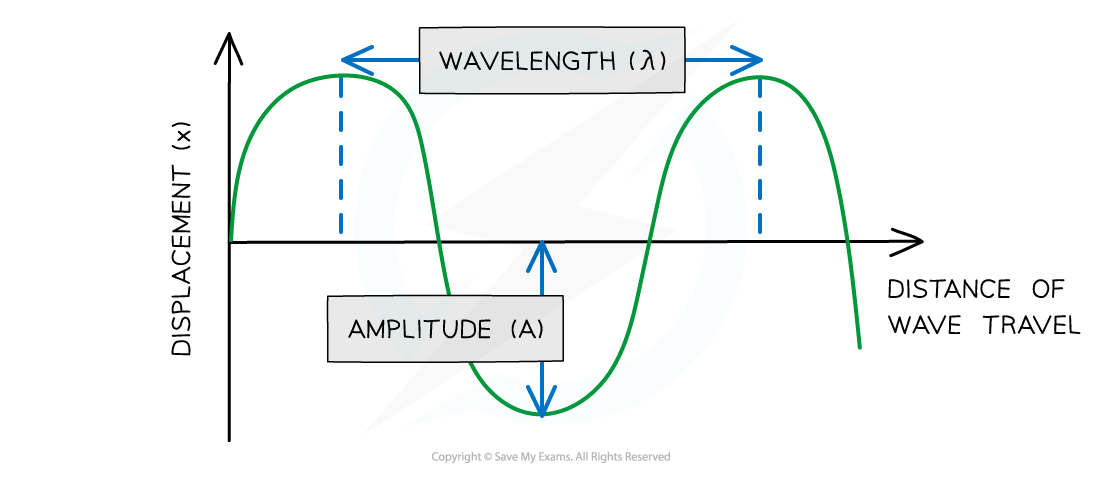Diagram showing the amplitude and wavelength of a wave

• The frequency, f, and the period, T, of a travelling wave are related to each other by the equation: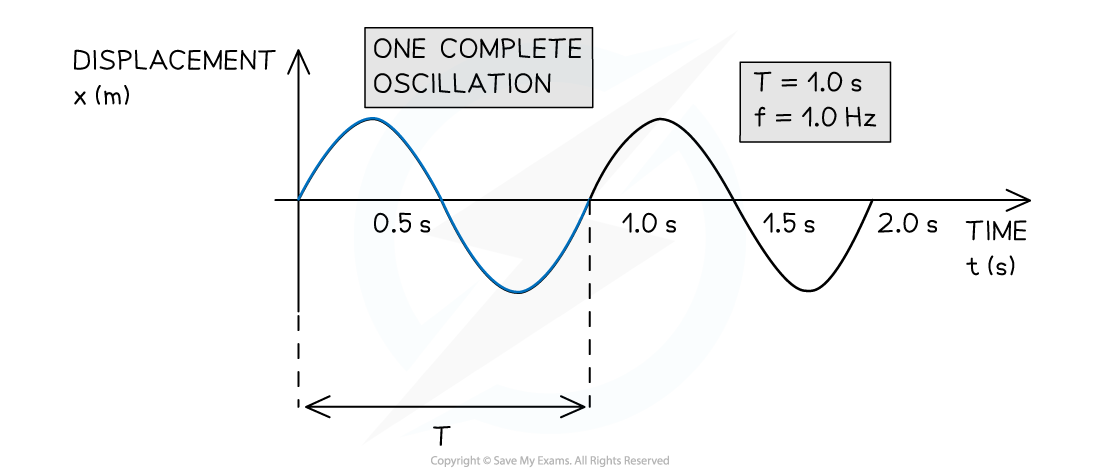Period T and frequency f of a travelling wave

#### Worked Example

The graph below shows a travelling wave.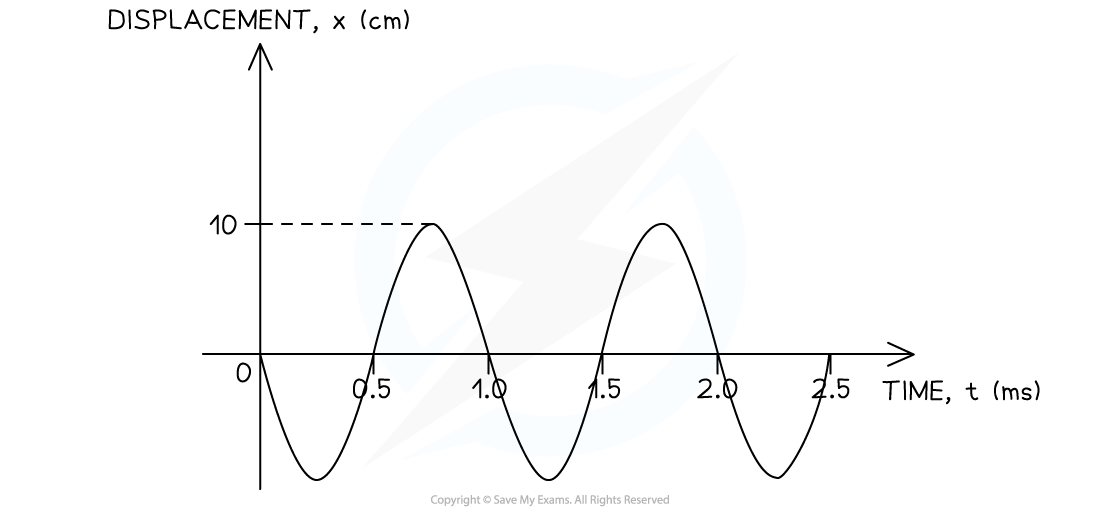Determine:

(i) The amplitude A of the wave in metres (m)

(ii) The frequency f of the wave in hertz (Hz)

(i) Identify the amplitude A of the wave on the graph

• The amplitude is defined as the maximum displacement from the equilibrium position (x = 0)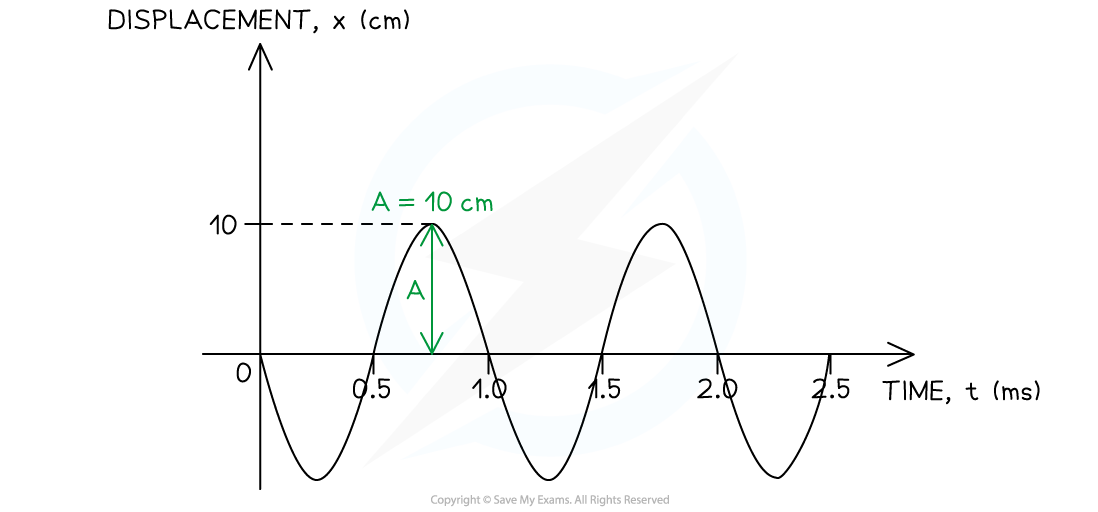• The amplitude must be converted from centimetres (cm) into metres (m)
A = 0.1 m

(ii) Calculate the frequency of the wave

Step 1: Identify the period T of the wave on the graph

• The period is defined as the time taken for one complete oscillation to occur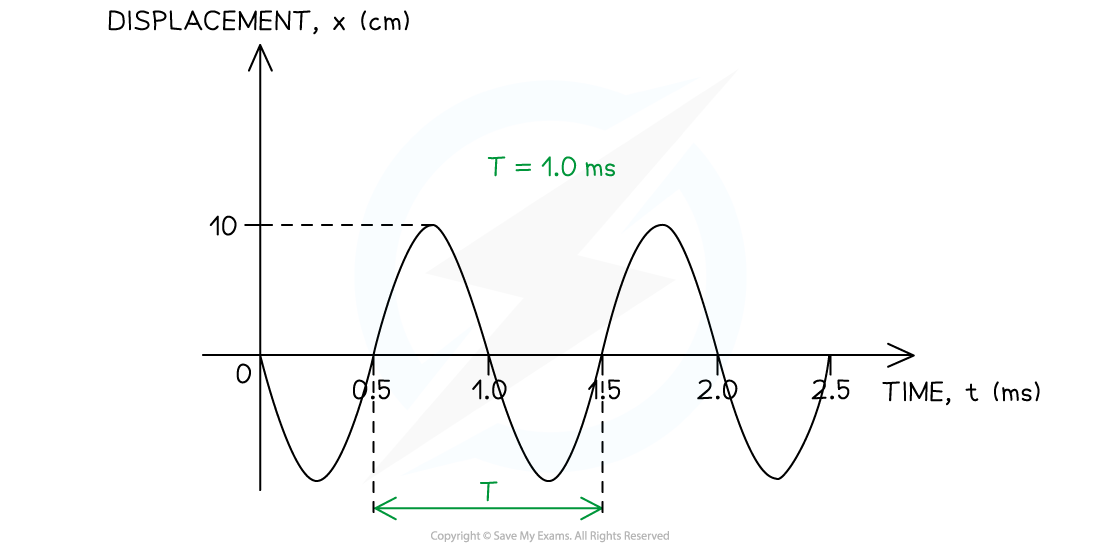• The period must be converted from milliseconds (ms) into seconds (s)

T = 1 × 10–3 s

Step 2: Write down the relationship between the frequency f and the period T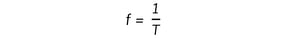Step 3: Substitute the value of the period determined in Step 1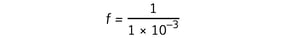f = 1000 Hz

### The Wave Equation

• The wave equation describes the relationship between the wave speed, the wavelength and the frequency of the wave

c = fλ

• Where
• c = wave speed in metres per second (m s−1)
• f = frequency in hertz (Hz)
• λ = wavelength in metres (m)

#### Deriving the Wave Equation

• The wave equation can be derived using the equation for speed
•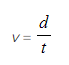Where
• v = velocity or speed in metres per second (m s−1)
• d = distance travelled in metres (m)
• t = time taken in seconds (s)
• When the source of a wave undergoes one complete oscillation, the travelling wave propagates forward by a distance equal to one wavelength λ
• The travelling wave covers this distance in the time it takes the source to complete one oscillation, the time period T
•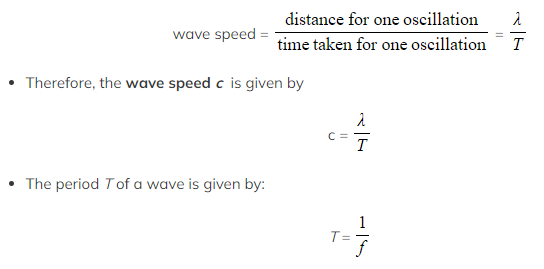Therefore, combining these equations gives the wave equation

c = fλ

#### Worked Example

A travelling wave has a period of 1.0 μs and travels at a velocity of 100 cm s–1. Calculate the wavelength of the wave. Give your answer in metres (m).

Step 1: Write down the known quantities

• Period, T = 1.0 μs = 1.0 × 10–6 s
• Velocity, c = 100 cm s–1 = 1.0 m s–1

Note the conversions:

• The period must be converted from microseconds (μs) into seconds (s)
• The velocity must be converted from cm s–1 into m s–1

Step 2: Write down the relationship between the frequency f and the period TStep 3: Substitute the value of the period into the above equation to calculate the frequency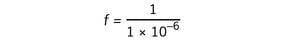f = 1.0 × 106 Hz

Step 4: Write down the wave equation

c = fλ

Step 5: Rearrange the wave equation to calculate the wavelength λ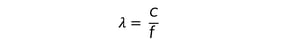Step 6: Substitute the numbers into the above equation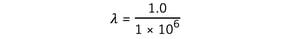λ = 1 × 10–6 m